# 一对多数据查询，学会套路才简单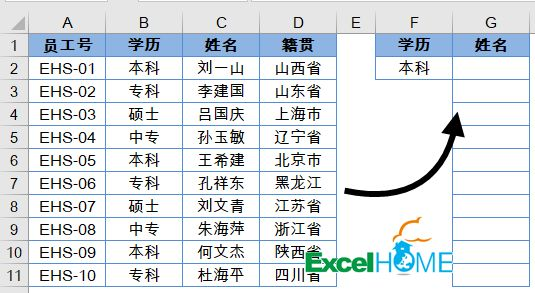A~D列是一些员工信息，要根据F2单元格指定的学历，提取出所有“本科”的人员姓名。

G2单元格输入以下公式，按住Shift+ctrl不放，按回车，再将公式向下拖动到出现空白单元格为止：

``=INDEX(C:C,SMALL(IF(B\$2:B\$11=F\$2,ROW(\$2:\$11),4^8),ROW(A1)))&""``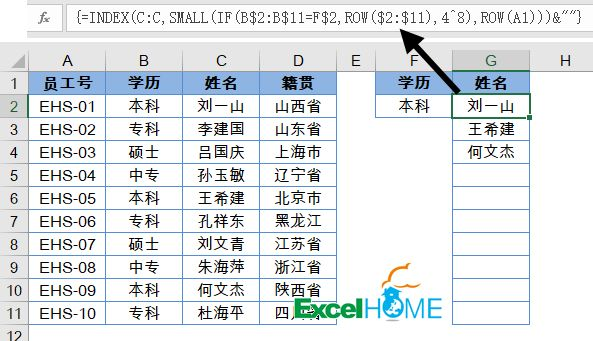``IF(B\$2:B\$11=F\$2,ROW(\$2:\$11),4^8)``

IF函数的意思是判断一个条件是不是成立，如果成立返回第二参数，否则返回第三参数。

{2;65536;65536;65536;6;65536;65536;65536;10;65536}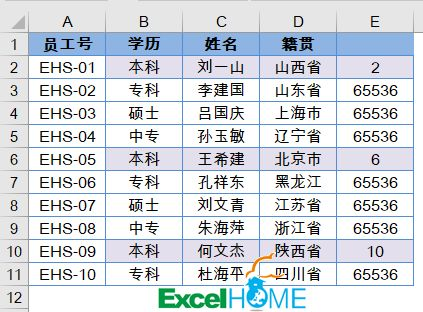SMALL函数的作用是返回一组数值中的第n个最小值，比如公式SMALL(A:A,3)，就是返回A列中的第三个最小值了。

ROW(A1)的作用是返回A1单元格的行号，结果是1。当公式向下复制时，参数会依次变成ROW(A2)、ROW(A3)、……，也就是得到从1开始、依次递增的序号。最终的目的是给SMALL函数一个动态的参数，依次从内存数组中提取出第1至n个最小值。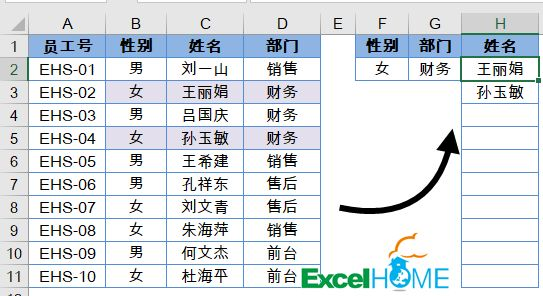H2单元格输入以下公式，按住Shift+ctrl不放，按回车，再将公式向下拖动到出现空白单元格为止：

``=INDEX(C:C,SMALL(IF((\$B\$2:\$B\$11=\$F\$2)*(\$D\$2:\$D\$11=\$G\$2),ROW(\$2:\$11),4^8),ROW(A1)))&""``

(\$B\$2:\$B\$11=\$F\$2)*(\$D\$2:\$D\$11=\$G\$2)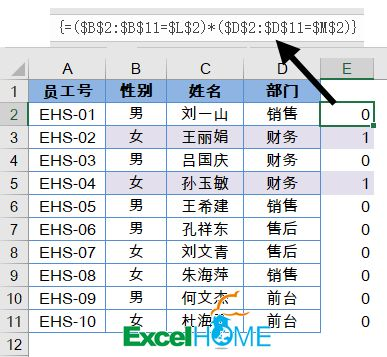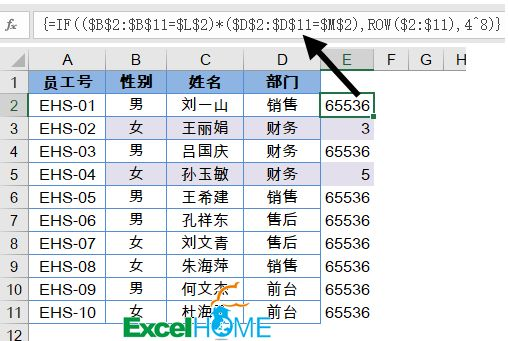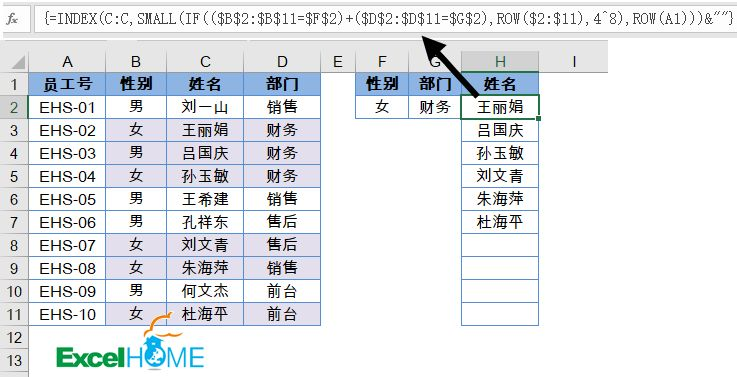``=INDEX(C:C,SMALL(IF((\$B\$2:\$B\$11=\$F\$2)+(\$D\$2:\$D\$11=\$G\$2),ROW(\$2:\$11),4^8),ROW(A1)))&""``